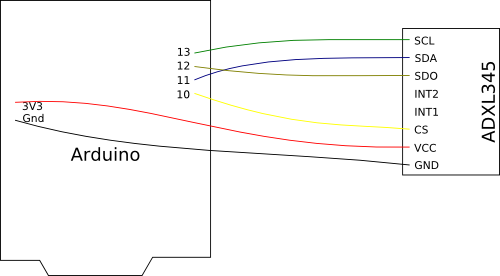+즐겨찾기    로그인   회원가입    고객센터블로그카페 코딩 교육 견적,대량요청 서류요청 후불결제 리셀러 베타리워즈
상품카테고리 > 전자 부품 > 센서보드 > IMU센서아두이노 호환 디지털 가속도 센서 모듈 (ADXL345 Digital Angle acceleration sensor module)판매가격 : 2,530원 (부가세 포함) MIZ-B111-ZAS
배송일 : 당일발송 OEM 40개 이상개총 금액 :

 상품상세 관련상품 배송/교환/반품 안내 상품리뷰ADXL345는 작고 얇고, 저전력 3축 가속도센서로 고분해능 (13비트)을 가지고 있습니다. 충격 측정 및 방향 센싱을 할 수 있습니다.

2.0-3.6VDC Supply Voltage
Ultra Low Power: 40uA in measurement mode, 0.1uA in standby@ 2.5V
SPI and I2C interfaces
Free-Fall Detection

데이터시트

사용 예제

 Arduino Pin ADXL345 Pin 10 CS 11 SDA 12 SDO 13 SCL 3V3 VCC Gnd GND//Add the SPI library so we can communicate with the ADXL345 sensor
#include

//Assign the Chip Select signal to pin 10.
int CS = 10;

//This is a list of some of the registers available on the ADXL345.
char POWER_CTL = 0x2D;  //Power Control Register
char DATA_FORMAT = 0x31;
char DATAX0 = 0x32; //X-Axis Data 0
char DATAX1 = 0x33; //X-Axis Data 1
char DATAY0 = 0x34; //Y-Axis Data 0
char DATAY1 = 0x35; //Y-Axis Data 1
char DATAZ0 = 0x36; //Z-Axis Data 0
char DATAZ1 = 0x37; //Z-Axis Data 1

unsigned char values;
//These variables will be used to hold the x,y and z axis accelerometer values.
int x, y, z;
int lastx, lasty, lastz;
int change;
double xg, yg, zg;
void setup() {
//Initiate an SPI communication instance.
SPI.begin();
//Configure the SPI connection for the ADXL345.
SPI.setDataMode(SPI_MODE3);
//Create a serial connection to display the data on the terminal.
Serial.begin(9600);

//Set up the Chip Select pin to be an output from the Arduino.
pinMode(CS, OUTPUT);
//Before communication starts, the Chip Select pin needs to be set high.
digitalWrite(CS, HIGH);

//Put the ADXL345 into +/- 4G range by writing the value 0x01 to the DATA_FORMAT register.
writeRegister(DATA_FORMAT, 0x00);
//Put the ADXL345 into Measurement Mode by writing 0x08 to the POWER_CTL register.
writeRegister(POWER_CTL, 0x08);  //Measurement mode
}

void loop() {
//Reading 6 bytes of data starting at register DATAX0 will retrieve the x,y and z acceleration values from the ADXL345.
//The results of the read operation will get stored to the values[] buffer.

//The ADXL345 gives 10-bit acceleration values, but they are stored as bytes (8-bits). To get the full value, two bytes must be combined for each axis.
//The X value is stored in values and values.
x = ((int)values << 8) | (int)values;
//The Y value is stored in values and values.
y = ((int)values << 8) | (int)values;
//The Z value is stored in values and values.
z = ((int)values << 8) | (int)values;
xg = x * 0.0078;
yg = y * 0.0078;
zg = z * 0.0078;
change = abs(lastx - x) + abs(lasty - y) + abs(lastz - z);
lastx = x;
lasty = y;
lastz = z;
//Print the results to the terminal.
//    Serial.print((float)xg,2);
//      Serial.print("g,");
//      Serial.print((float)yg,2);
//      Serial.print("g,");
//      Serial.print((float)zg,2);
//      Serial.println("g");
//if(change > 4) {
//    Serial.print("Change:");
Serial.println(change);
//}
delay(10);
}

//This function will write a value to a register on the ADXL345.
//Parameters:
//  char registerAddress - The register to write a value to
//  char value - The value to be written to the specified register.
void writeRegister(char registerAddress, char value) {
//Set Chip Select pin low to signal the beginning of an SPI packet.
digitalWrite(CS, LOW);
//Transfer the register address over SPI.
//Transfer the desired register value over SPI.
SPI.transfer(value);
//Set the Chip Select pin high to signal the end of an SPI packet.
digitalWrite(CS, HIGH);
}

//This function will read a certain number of registers starting from a specified address and store their values in a buffer.
//Parameters:
//  int numBytes - The number of registers that should be read.
//  char * values - A pointer to a buffer where the results of the operation should be stored.
//Since we're performing a read operation, the most significant bit of the register address should be set.
//If we're doing a multi-byte read, bit 6 needs to be set as well.

//Set the Chip select pin low to start an SPI packet.
digitalWrite(CS, LOW);